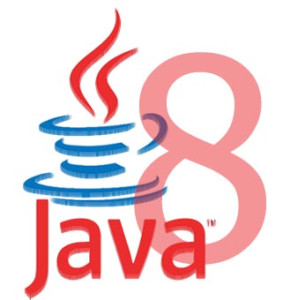21.What is the purpose of IntSupplier functional interface?
It represents a supplier of int-valued results.

22.What is the purpose of IntToDoubleFunction functional interface?
It represents a function that accepts an int-valued argument and produces a double-valued result.

23.What is the purpose of IntToLongFunction functional interface?
It represents a function that accepts an int-valued argument and produces a long-valued result.

24.What is the purpose of IntUnaryOperator functional interface?
It represents an operation on a single int-valued operand that produces an int-valued result.

25.What is the purpose of LongBinaryOperator functional interface?
It represents an operation upon two long-valued operands and produces a long-valued result.

26.What is the purpose of LongConsumer functional interface?
It represents an operation that accepts a single long-valued argument and returns no result.

27.What is the purpose of LongFunction<R> functional interface?
It represents a function that accepts a long-valued argument and produces a result.

28.What is the purpose of LongPredicate functional interface?
It represents a predicate (Boolean-valued function) of one long-valued argument.

29.What is the purpose of LongSupplier functional interface?
It represents a supplier of long-valued results.

30.What is the purpose of LongToDoubleFunction functional interface?
It represents a function that accepts a long-valued argument and produces a double-valued result.